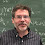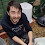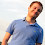## Tuesday, April 04, 2017

### Proving Langs not Regular using Comm Complexity

(My notes on this are at my course website: here They are notes for my ugrad students so they may be longer and more detailed than you want.)

While Teaching Regular langauges in the Formal Languages course I realized

Using that  { (x,y) : x=y, both of length n}  has Communication Complexity \ge n+1 one can easily prove:

a) The Language \{ xx : x\in \Sigma^*} is NOT regular

b) For all n the language \{ xx : x \in \Sigma^n }, which is regular, requires a DFA on 2^{n+1} states.

I also used Comm Complexity to show that

{ w : the number of a's in w is a square}  is not regular, from which one can get

{ a^{n^2} : n\in N} is not regular.

More generally, if A is any set such that there are arb large gaps in A, the set

{ w : the number of a's in w is in A} and {a^n : n \in A} are not regular.

This approach HAS TO BE KNOWN and in fact it IS- Ian Glaister and Jeffrey Shallit had a paper in 1996 that gave lower bounds on the size of NFA's using ideas from Comm Complexity (see here). They present  their technique as a way to get  lower bounds on the size of NFA's; however, their techniques  can easily be adapted to get all of the results I have, with similar proofs to what I have.
(Jeffrey Shallit, in the comments,  pointed me to an article that predates him that had similar ideas:here.)
(Added later- another early  referene on applying comm comp to proving langs not regular is Communication Complexity. Advances in Computers Vol 44 Pages 331-360 (1997),
section 3.1, by Eyal Kushlevitz. (See here)

Next time you teach Automata theory you may want to teach showing langs are NOT regular using Comm Complexity. Its a nice technique that also leads to lower bounds on the number of states for DFA's and NFA's.

1.Turns out our ideas weren't new; they appeared in this paper: J.-C. Birget. Intersection and union of regular languages and state complexity.
Inform. Process. Lett. 43 (1992), 185-190.

1.Thanks. I have added this ref to the post.

2.Arguably, Regular languages are simpler to understand than Communication complexity, and are thought earlier, that's why these proofs are ot used. Otherwise, later the proof that Palindromes cannot be decided on a single-tape Turing-machine in o(log n) space (in fact not even o(n^2) time) also uses essentially the same idea.

3.I recently asked references for a very related technique here: http://cstheory.stackexchange.com/questions/37136/regular-languages-and-constant-communication-complexity

I note there that Hauser has a general technique for 2-player communication complexity when the input is spread in a finite number of blocks between Alice and Bob.

A different approach, with multiple players and a fine study of the complexity of the resulting language, is given by:

A. Chattopadhyay, A. Krebs, M. Kouckỳ, M. Szegedy, P. Tesson, and D. Thérien, “Languages with bounded multiparty communication complexity,” in Annual Symposium on Theoretical Aspects of Computer Science. Springer, 2007, pp. 500–511.

and the citations therein.

To add a bit of self-advertising, in https://arxiv.org/abs/1701.02673 we use a simple communication complexity technique to show that a language is in MSO[<] augmented with all monadic numerical predicates. To do so, we adapt the folklore proof of my above CSTheory question.

Cheers!

4.Is this technique stronger than the pumping lemma?

1.I believe this is open. Here is phrasing of it, both directions
1) Is there a nonreg L such that
- there is a constant c such that for all w\in L, |w|\ge c,
there exists x,y,z w=xyz with |xy|\le c such that
xy*z is in L. AND comm comp of { (x,y): |x|=|y|=n and xy\in L} is nonconstnat.
2) Is there a non-reg lang L such that comm comp of
{ (x,y) : |x|=|y|=n and xy\in L} is O(1) but pumping does prove its not regular. Actually this a a YES but with a silly example that I would want to not allow

{ xy : |x|=|y| and x has same number of a's as b's}

5.I there a (known) way to rephrase the classical proof that palindromes cannot be recognized in time o(n²) on a 1-tape TM using communication complexity? I am aware that the classical proof has a flavor of communication complexity. My question is: Is it possible to use a lower bound on the deterministic communication complexity of {(x,y):|x|=|y|=n and x=ȳ}, where ȳ is the mirror of y, to derive the lower bound (or some lower bound) on the 1-tape complexity of palindromes? I guess that the deterministic communication complexity of the set I defined is n+1 (same proof as for equality), but I was unable to adapt the proof you present for {xx:x in {0,1}*} not being regular to my case.

6.@B. It's in Section 12.2 of the Kushilevitz-Nisan book. The main claim is that, given a function f:{0,1}^n \times {0,1}^n \to {0,1}, the time complexity of recognizing the language L_f = {x0^{n}y: f(x,y) = 1} on a single tape TM is at least n*R_0(f), where R_0 is randomized zero-error communication complexity (with public randomness). This gives a quadratic lower bound for recognizing palindromes using a communication lower bound for equality.

For the argument, fix a single tape TM that recognizes L_f in time T(n). We will use it to give a zero error protocol with expected complexity O(T(n)/n). Alice and Bob pick an index i uniformly at random from {n+1, …, 2n}. This is the index of one the n zeros in the middle of the input. Then Alice and Bob together simulate the TM basically in the obvious way: whenever the head is to the left of i Alice does the simulation, and when the tape crosses over to the right of i she sends the internal state to Bob, and then he continues the simulation, and the same happens in reverse when the head crosses over from the right of i to the left. Each crossing costs constant communication, and the expected number of times the head crosses over i is at most T(n)/n.

7.Myhill-Nerode is very much in the spirit of these communication complexity arguments and it may be nice to teach it that way. If I am not missing something, you can restate the theorem as "the deterministic one-way communication complexity of L is O(1) if and only if L is regular". Here the communication problem associated with a language is for Alice and Bob to determine if their concatenated inputs are in L.

1.Not iff. What if L is { xy : |x|=|y| and x\in HALT}
Then the Comm Comp of L is O(1) but L is not decidable.
We need some way to ban these kinds of contrived counterexamples.

2.I am not sure this is O(1) under the definition of a communication problem I was trying to describe. In the communication problem for language L, Alice receives an string x of arbitrary length, and Bob received another string y also of arbitrary length. Alice sends a single message to Bob, who must use the message and y to decide if xy \in L. I can see how for the language you define the communication complexity is O(1) if Alice and Bob each get half of the word whose membership needs to be decided. But in my definition I allow uneven splits, and I don't think the communication complexity will be O(1) if Alice doesn't get all of x.

3.Uneven splits- interesting idea but could mean a few things:
Fix n
Comm Complexity is max over all splits of x,y
min?

4.I've taught some basics of communication complexity alongside Myhill-Nerode (and streaming algorithms) for a number of years now in my undergrad automata theory course. (The slides are available online from my webpage.)

What Sasho is describing is related to a homework exercise in the same course :) Under the model being described where Alice and Bob get arbitrary splits of a string into halves, and must get O(1) communication for every split, two-way communication should also work (because two way DFAs = DFAs)

5.I agree there is ambiguity in what communication complexity means for uneven splits. I think the complexity of words of size n should be max over all words of size n and all ways to split them. Or it may also make sense to measure complexity with respect to the size of Alice's input only, since this is a one-way model. It seems that since we only care about O(1) complexity, the finer details are not crucial.

8.@Sasho: Thanks! I could have a look at Kushilevitz and Nisan's book that is on my shelf... The proof is really close to the classical one since it uses, though in some slightly disguised form, the notion of crossing sequence.

1.Crossing sequences are a special case of communication complexity.

9.DFA size and deterministic communication complexity of a language in a uniform model of one-way communication are equivalent.

The uniform model is that Alice gets a string x and Bob a string y, and the protocol has to decide if xy is in the language.

Once you change to non-deterministic or randomized the connection breaks down (lower bounds for automata size remain valid).

http://citeseerx.ist.psu.edu/viewdoc/summary?doi=10.1.1.28.4448

contains some attempts to use this connection for non-deterministic automata.

10.What is "Langs", and what is "Comm"? Are these special concepts in complexity?

1.AH-you raise the good point that after a while a community is so used to certain abbreviations that they (or at least me) assumes they are well known.

Langs means Languages. For us a Language is a set of strings. What is a string? A string is a sequence of characters in an alphabet that we all agree on. If the alphabet is {a,b} then
aabbbaaa would be a string.

Comm means Communication.

Are these special in complexity?
Alphabet, string, Language are common in all of Computer science, though Language may be something else to people who work in Programming Languages. Langs for Languages is probably not standard, though I suspect most people reading this column knew what I meant (thank YOU for keeping me honest!). Comm for Communication- not sure if thats common.